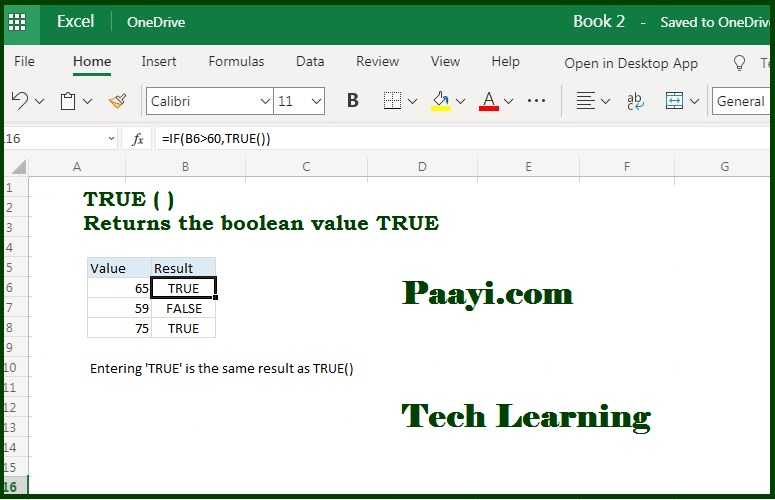# Learn How to Use Microsoft Excel TRUE Function

Written by | 0 Comments | 743 Views

In this article, you will learn how to use the Microsoft Excel TRUE function and its prime function in Microsoft Excel. You will also get to know the Microsoft Excel TRUE function return value and syntax with the help of some examples.

## Microsoft Excel TRUE Function

The TRUE function generates the TRUE value. It means the Microsoft Excel TRUE function will always return the TRUE value. It is one of the compatibility functions, and it is not usually required in most of the Microsoft Excel functionality. You can consider it as similar to the Boolean TRUE value directly in a formula.

### The TRUE Function Return Value

It will return the logical value TRUE.

=TRUE( )

## How to Use Microsoft Excel TRUE Function?There is no need to use the TRUE function in the standard applications, and it is provided for compatibility with the other Microsoft Excel applications. You can use the TRUE function directly by just entering the TRUE in the formula, and Microsoft Excel will interpret it as a logical value TRUE.